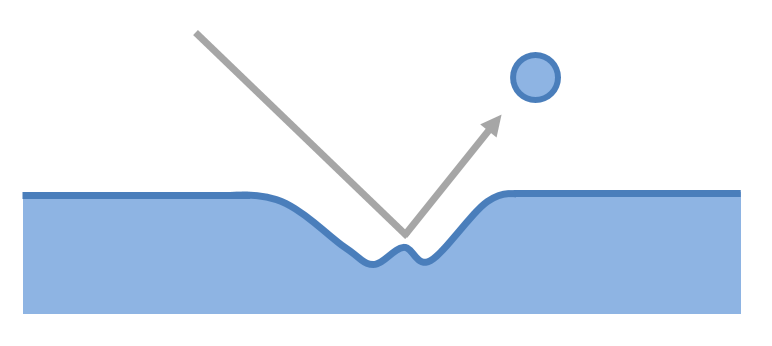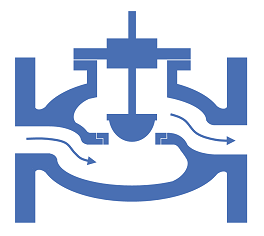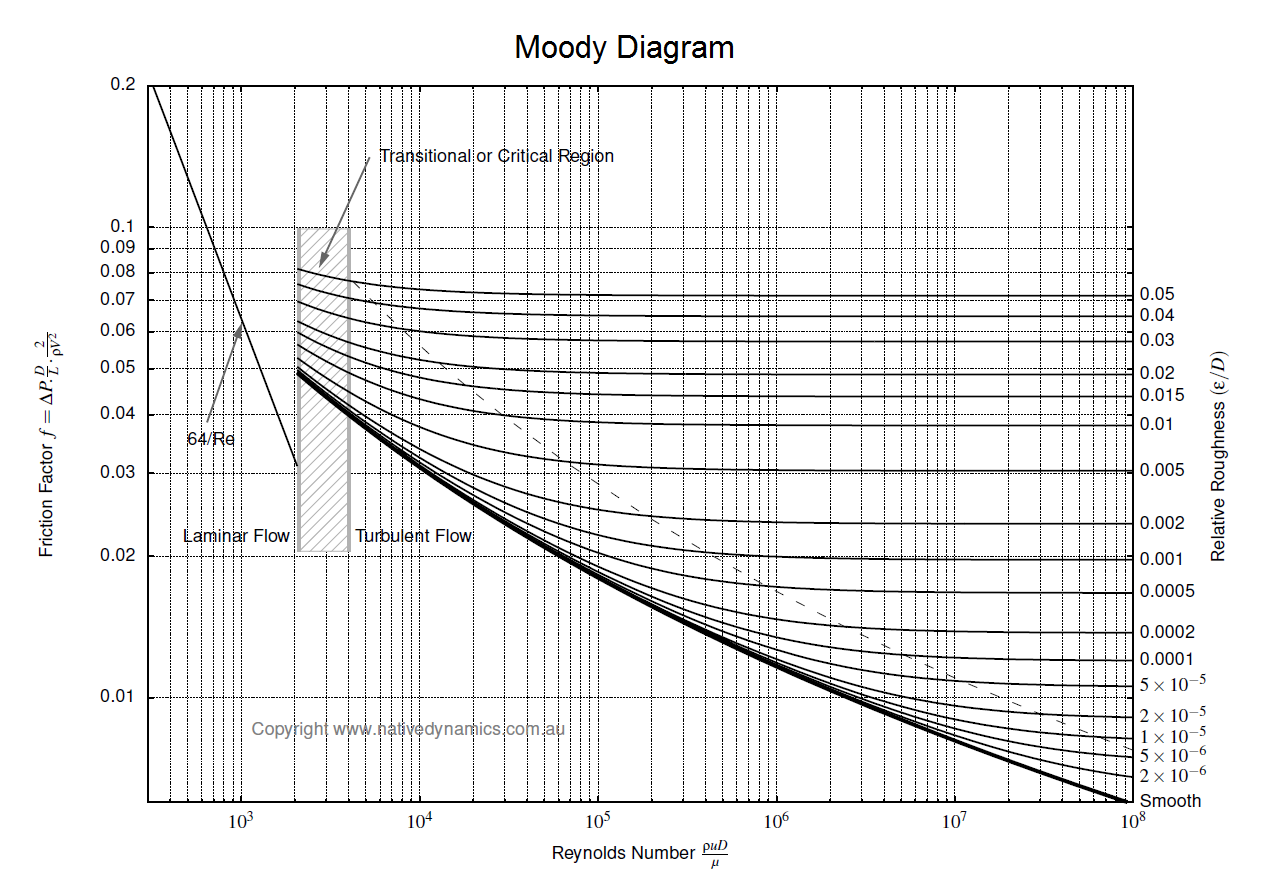f
Neutrium

# Pipe

Erosion Velocity for Gas-Liquid Multiphase FlowThe flow of a gas-liquid multiphase system may cause erosion if velocities are high. This article presents an empirical relationship for estimating whether erosion will occur in a system at a certain velocity.

Speed of Sound in Fluids and Fluid in Pipes

This article provides the formulae for the calculation of speed of sound in fluids and fluid filled circular pipes. The speed of sounds is important in piping systems for the calculation of choked flow for gases and pressure transient analysis of liquid filled systems.

Birmingham Wire Gauge

Birmingham Wire Gauge (BWG), also known as Stubs Iron Wire Gauge is a system of measurements describing thickness. BWG has been historically used to describe the outside diameter of small tubes and wires, as well as the wall thickness of some large tubes (such as those used within a shell and tube heat exchanger). This article presents length conversions for BWG values into metric and imperial units.

Nominal Pipe Characteristics in Metric Units

Nominal Pipe Size (NPS) is a defined set of standard pipe sizes commonly used for process piping. NPS piping is defined in terms of a nominal diameter and wall thickness (defined by the pipe schedule). This article provides characteristics for NPS piping in metric units.

Nominal Pipe Characteristics in Imperial Units

Nominal Pipe Size (NPS) is a defined set of standard pipe sizes commonly used for process piping. NPS piping is defined in terms of a nominal diameter and wall thickness (defined by the pipe schedule). This article provides characteristics for NPS piping in Imperial units.

Converting Between Cv, Kv and KThere are several common ways to express the losses caused by pipe fittings and equipment. Depending on the calculation programs or methods available and engineer may require to convert between one form or another. This article details the equations required to convert between the resistance coefficient and flow coefficient methods (K, Cv and Kv).

Pressure Loss in PipeTo determine the pressure loss or flow rate through pipe knowledge of the friction between the fluid and the pipe is required. This article describes how to incorporate friction into pressure loss or fluid flow calculations. It also outlines several methods for determining the Darcy friction factor for rough and smooth pipes in both the turbulent and laminar flow regime. Finally this article discusses which correlation for pressure loss in pipe is the most appropriate.